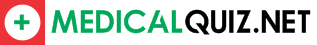# Psychology Vocabulary Quiz

Preview:

a statement that is the opposite of the original hypothesis. Research must be compared to the null hypothesis (the opposite scenario) to see if any variance is in fact due to the independent variable

A. Null hypothesis

B. hypothesis

C. Inferential statistics

D. Overconfidence

the post experimental explanation of a study, including its purpose and any deceptions, to its participants

A. Descriptive statistic

B. Hawthorne effect

C. Debrief

D. Median

a statistical index of the relationship between two things (from -1 to +1)

A. Experimental group

B. Statistical significance

C. Correlation coefficient

D. Inferential statistics

in an experiment when neither the participants nor the researchers know who belongs to the control group and who belongs to the experimental group. Only after all the data have been recorded (and in some cases, analyzed) do the researchers learn which individuals are which.

A. Single-blind procedure

B. Inferential statistics

C. Double-blind procedure

D. Measure of central tendency

established by the A.P.A, guidelines that outline what s acceptable and unacceptable for treatment of participants in research studies

A. Range

B. Ethics

C. Skewed distribution

D. Theory

the middle score in a distribution, half the scores are above it and half are below it

A. mean

B. range

C. median

D. mode

an ethical principle the research participants’ identity and information remain private in any research study

A. Confidentiality

B. Overconfidence

C. Theory

D. Validity

an ethical principle that research participants be told enough to enable them to choose whether they wish to participate

A. Ethics

B. Single-blind procedure

C. Informed consent

D. Uniformed Consent

in an experiment, the group that is not exposed to the treatment: contrast with the experimental group ad serves as a comparison for evaluation the effects of the treatment

A. Debrief

B. Experimental group

C. Control group

D. Inferential statistics

refers to how spread apart the scores of the distribution are or how much the scores vary from each other. There are four major measures of variability, including the range, interquartile range variance, and standard deviation

A. Measure of central tendency

B. Measures of variation

C. Null hypothesis

D. Operational definitions

the outcome factor; the variable that may change in response t manipulations of the independent variable

A. Independent variable

B. Dependent variable

C. Histogram

techniques that allow researchers to make generalizations (or to infer) about the populations from which the samples were drawn.

A. Inferential statistics

B. Normal distribution curve

C. Descriptive statistic

D. Operational definition

a statement of procedures (operations) used to define research variables. For example, human intelligence may be operationally defined as what an intelligence test measures. The operational definition must explain how the variable will be measured.

A. Debrief

B. Operational definitions

C. Experiment

the arithmetic average of a distribution, obtained by adding the scores then dividing by the number of scores

A. Range

B. Mode

C. Median

D. Mean

a factory other than the independent variable that might produce an effect in an experiment

A. Operational definition

B. Independent variable

C. Measures of variation

D. Confounding variables

when patients given a placebo treatment will have a perceived or actual improvement in a medical condition

A. Random assignments

B. placebo effect

C. placebo

D. Replicate

the tendency to overestimate how easy something might be or how long a task might take

A. overconfidence

B. Placebo effect

C. population

D. Replicate

the most frequently occurring score(s), in a distribution

A. mean

B. median

C. mode

D. range

When the researcher himself alters or changes the result of the study. For example, a teacher studying differences in math skills between boys and girls might spend more time teaching boys because he/she believes that boys are better at math

A. Operational definitions

B. Overconfidence

C. Observer bias

D. Random sample

a simulated or otherwise medically ineffectual (fake) treatment for a disease or other medical condition intended to deceive the recipient. Often given to the control group in an experiment.

A. Scatterplot

B. Placebo effect

C. Skewed distribution

D. Placebo

a group of procedures that summarize or describe a set of data. These procedures include the measurement of central tendency and measures of variability

A. Inferential statistics

B. Descriptive statistic

C. Illusory correlation

D. Measure of central tendency

in an experiment, the group that is exposed to the treatment, that is, to one version of the independent variable

A. Statistical significance

B. Experimental group

C. Inferential statistics

D. Measure of central tendency

All the cases in a group being studied, from which samples may be drawn

A. Population

B. Random sample

C. Random assignments

D. Sampling bias

a measure of the extent to which two factors vary together, and thus of how well either factor predicts the other

A. Correlation

B. Correlation coefficient

C. Naturalistic observations

D. Statistical significance

the perception of a relationship where none exist

A. Independent variable

B. Illusory correlation

C. Inferential statistics

D. Measure of central tendency

Disclaimer:

Medical Quiz should not be considered complete, up to date, and is not intended to be used in place of a visit, consultation, or advice of a legal, medical, or any other professional. All content on this website is for informational and educational purposes only.